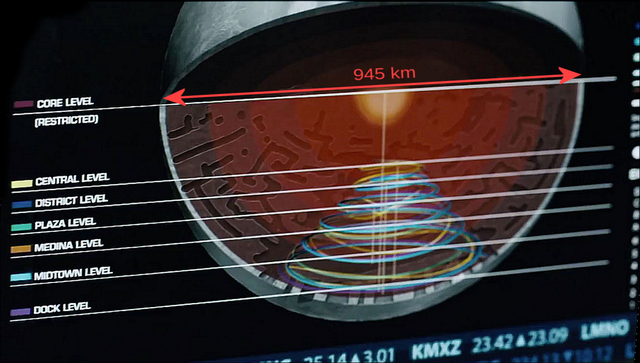## June 14, 2016

### Coriolis

I really like the science fiction TV series The Expanse. In addition to a good plot and a convincing vision of human society two centuries hence, it depicts, as Phil Plait observes, a lot of good science in a matter-of-fact, almost off-hand fashion. But one scene (really, just a few dialogue-free seconds in a longer scene) has been bothering me. In it, Miller, the hard-boiled detective living on Ceres, pours himself a drink. And we see — as the whiskey slowly pours from the bottle into the glass — that the artificial gravity at the lower levels (where the poor people live) is significantly weaker than near the surface (where the rich live) and that there’s a significant Coriolis effect. Unfortunately, the effect depicted is 3 orders-of-magnitude too big.To explain, six million residents inhabit the interior of the asteroid, which has been spun up to provide an artificial gravity. Ceres has a radius, $R_C = 4.73\times 10^5$ m and a surface gravity $g_C=.27\,\text{m}/\text{s}^2$. The rotational period is supposed to be 40 minutes ($\omega\sim 2.6\times 10^{-3}\, /\text{s}$). Near the surface, this yields $\omega^2 R_C(1-\epsilon^2)\equiv \omega^2 R_C -g_C \sim 0.3$ g. On the innermost level, $R=\tfrac{1}{3} R_C$, and the effective artificial gravity is only 0.1 g.So how big is the Coriolis effect in this scenario?

The equations1 to be solved are

(1)$\begin{split} \frac{d^2 x}{d t^2}&= \omega^2(1-\epsilon^2) x - 2 \omega \frac{d y}{d t}\\ \frac{d^2 y}{d t^2}&= \omega^2(1-\epsilon^2) (y-R) + 2 \omega \frac{d x}{d t} \end{split}$

with initial conditions $x(t)=\dot{x}(t)=y(t)=\dot{y}(t)=0$. The exact solution solution is elementary, but for $\omega t\ll 1$, i.e. for times much shorter than the rotational period, we can approximate

(2)$\begin{split} x(t)&= \frac{1}{3} (1-\epsilon^2) R (\omega t)^3 +O\bigl((\omega t)^5\bigr),\\ y(t)&= - \tfrac{1}{2} (1-\epsilon^2)R(\omega t)^2+O\bigl((\omega t)^4\bigr) \end{split}$

From (2), if the whiskey falls a distance $h\ll R$, it undergoes a lateral displacement

(3)$\Delta x = \tfrac{2}{3} h\, {\left(\frac{2h}{(1-\epsilon^2)R}\right)}^{1/2}$

For $h=16$ cm and $R=\tfrac{1}{3}R_C$, this is $\frac{\Delta x}{h}= 10^{-3}$ which is 3 orders of magnitude smaller than depicted in the screenshot above2.

So, while I love the idea of the Coriolis effect appearing — however tangentially — in a TV drama, this really wasn’t the place for it.

1 Here, I’m approximating Ceres to be a sphere of uniform density. That’s not really correct, but since the contribution of Ceres’ intrinsic gravity to (3) is only a 5% effect, the corrections from non-uniform density are negligible.

2 We could complain about other things: like that the slope should be monotonic (very much unlike what’s depicted). But that seems a minor quibble, compared to the effect being a thousand times too large.

Posted by distler at June 14, 2016 4:40 PM

TrackBack URL for this Entry:   https://golem.ph.utexas.edu/cgi-bin/MT-3.0/dxy-tb.fcgi/2889

### Re: Coriolis

I read the books, and I don’t think they make that blunder in them, or at least I didn’t remember spotting it. Actually, I wonder what the biological effects of having a very large Coriolis force would be. If they were large as shown, I doubt the inner ear would be readily able to reach an equilibrium, especially if it was used to a certain levels effects. So transferring between layers would be a messy affair.

Posted by: Haelfix on June 15, 2016 11:18 PM | Permalink | Reply to this

### Re: Coriolis

What happened to that other post? Did you delete it? How come?

Posted by: Mitch on June 19, 2016 3:06 PM | Permalink | Reply to this

Post a New Comment